# augmenters.weather¶

Note

All examples below use the following input image: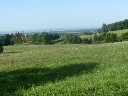## FastSnowyLandscape¶

Convert non-snowy landscapes to snowy ones.

This augmenter expects to get an image that roughly shows a landscape.

API link: `FastSnowyLandscape`

Example. Search for all pixels in the image with a lightness value in HLS colorspace of less than `140` and increase their lightness by a factor of `2.5`.

```import imgaug.augmenters as iaa
aug = iaa.FastSnowyLandscape(
lightness_threshold=140,
lightness_multiplier=2.5
)
```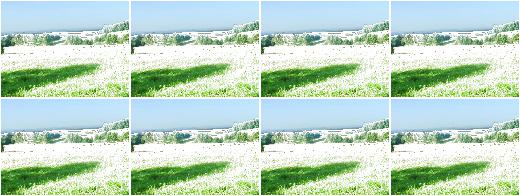Example. Search for all pixels in the image with a lightness value in HLS colorspace of less than `128` or less than `200` (one of these values is picked per image) and multiply their lightness by a factor of `x` with `x` being sampled from `uniform(1.5, 3.5)` (once per image).

```aug = iaa.FastSnowyLandscape(
lightness_threshold=[128, 200],
lightness_multiplier=(1.5, 3.5)
)
```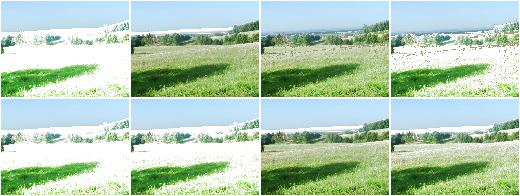Example. Similar to the previous example, but the lightness threshold is sampled from `uniform(100, 255)` (per image) and the multiplier from `uniform(1.0, 4.0)` (per image). This seems to produce good and varied results.

```aug = iaa.FastSnowyLandscape(
lightness_threshold=(100, 255),
lightness_multiplier=(1.0, 4.0)
)
```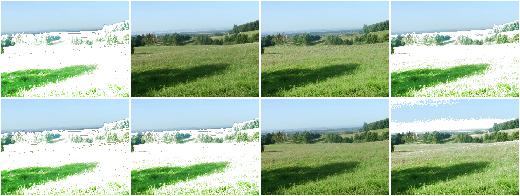## Clouds¶

This is a wrapper around `CloudLayer`. It executes 1 to 2 layers per image, leading to varying densities and frequency patterns of clouds.

This augmenter seems to be fairly robust w.r.t. the image size. Tested with `96x128`, `192x256` and `960x1280`.

API link: `Clouds()`

Example. Create an augmenter that adds clouds to images:

```import imgaug.augmenters as iaa
aug = iaa.Clouds()
```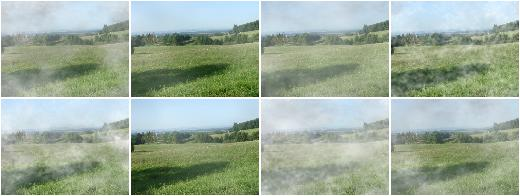## Fog¶

This is a wrapper around `CloudLayer`. It executes a single layer per image with a configuration leading to fairly dense clouds with low-frequency patterns.

This augmenter seems to be fairly robust w.r.t. the image size. Tested with `96x128`, `192x256` and `960x1280`.

API link: `Fog()`

Example. Create an augmenter that adds fog to images:

```import imgaug.augmenters as iaa
aug = iaa.Fog()
```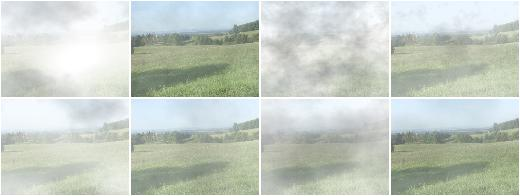## CloudLayer¶

Add a single layer of clouds to an image.

API link: `CloudLayer`

## Snowflakes¶

This is a wrapper around `SnowflakesLayer`. It executes 1 to 3 layers per image.

API link: `Snowflakes()`

Example. Add snowflakes to small images (around `96x128`):

```import imgaug.augmenters as iaa
aug = iaa.Snowflakes(flake_size=(0.1, 0.4), speed=(0.01, 0.05))
```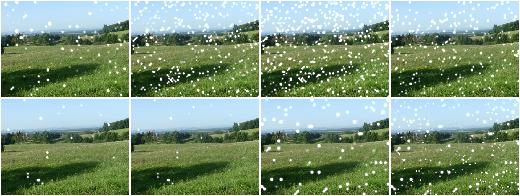Example. Add snowflakes to medium-sized images (around `192x256`):

```aug = iaa.Snowflakes(flake_size=(0.2, 0.7), speed=(0.007, 0.03))
```

Example. Add snowflakes to large images (around `960x1280`):

```aug = iaa.Snowflakes(flake_size=(0.7, 0.95), speed=(0.001, 0.03))
```

## SnowflakesLayer¶

Add a single layer of falling snowflakes to images.

API link: `SnowflakesLayer`

## Rain¶

This is a wrapper around `RainLayer`. It executes 1 to 3 layers per image.

Note

This augmenter currently seems to work best for medium-sized images around `192x256`. For smaller images, you may want to increase the speed value to e.g. `(0.1, 0.3)`, otherwise the drops tend to look like snowflakes. For larger images, you may want to increase the drop_size to e.g. `(0.10, 0.20)`.

API link: `Rain`

Example. Add rain to small images (around `96x128`):

```import imgaug.augmenters as iaa
aug = iaa.Rain(speed=(0.1, 0.3))
```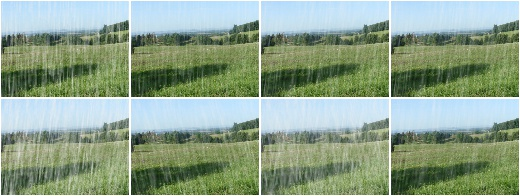Example. Add rain to medium sized images (around `192x256`):

```aug = iaa.Rain()
```

Example. Add rain to large images (around `960x1280`):

```aug = iaa.Rain(drop_size=(0.10, 0.20))
```

## RainLayer¶

Add a single layer of falling raindrops to images.

API link: `RainLayer`